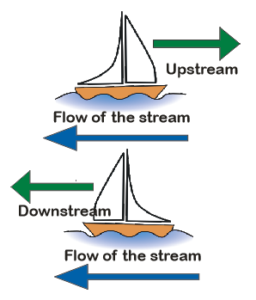d a boat in stream - - A boat goes 24 km downstream

# MCQ Word Problem 5 (Boat downstream upstream)

MCQ Word Problem 5 : A boat goes 24 km downstream in 10 hours, it takes 2 hours more to cover the same distance against the stream. What is the speed of the boat in still water?

a) 2 km/hr           b)2.2 km/hr         c) 4 km/hr       d) 4.8 km/hr

Solution:

This is a problem of a boat in a stream of running water. If boat goes with flow of water current then it is called going downstream. And if moving against the water current then it will be going upstream.Distance = speed x time

So,

$Speed = \frac {Distance} {time}$

In this way speed of boat downstream is:

$\frac{24}{10}= 2.4 \frac{km}{hr}$

Now since distance is same for upstream movement or against the stream –  but time is 2 hrs

So,  speed upstream is :

$\frac{24}{6}=2 \frac{km}{hr}$

Speed of the boat in still water is calculated by taking sum of downstream and upstream speeds and dividing it by 2.

So, speed in still water is:

$\frac{2+2.4}{2}=\frac{4.4}{2}=2.2 \frac{km}{hr}$

Hence the option  b is correct!